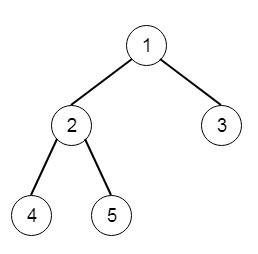# Diameter of Binary Tree in Python

Suppose we have a binary tree; we have to compute the length of the diameter of the tree. The diameter of a binary tree is actually the length of the longest path between any two nodes in a tree. This path not necessarily pass through the root. So if the tree is like below, then the diameter will be 3.as the length of the path [4,2,1,3] or [5,2,1,3] is 3To solve this, we will follow these steps −

• We will use the dfs to find the diameter, set answer := 0
• call the dfs function with the root dfs(root)
• dfs will work like below dfs(node)
• if node is not present, then return 0
• left := dfs(left subtree of root), and right := dfs(right subtree of root)
• return max of left + 1 and right + 1

## Example

Let us see the following implementation to get better understanding −

Live Demo

class TreeNode:
def __init__(self, data, left = None, right = None):
self.data = data
self.left = left
self.right = right
def insert(temp,data):
que = []
que.append(temp)
while (len(que)):
temp = que
que.pop(0)
if (not temp.left):
temp.left = TreeNode(data)
break
else:
que.append(temp.left)
if (not temp.right):
temp.right = TreeNode(data)
break
else:
que.append(temp.right)
def make_tree(elements):
Tree = TreeNode(elements)
for element in elements[1:]:
insert(Tree, element)
return Tree
class Solution(object):
def diameterOfBinaryTree(self, root):
"""
:type root: TreeNode
:rtype: int
"""
self.ans = 0
self.dfs(root)
return self.ans
def dfs(self, node):
if not node:
return 0
left = self.dfs(node.left)
right = self.dfs(node.right)
self.ans =max(self.ans,right+left)
return max(left+1,right+1)
root = make_tree([1,2,3,4,5])
ob1 = Solution()
print(ob1.diameterOfBinaryTree(root))

## Input

[1,2,3,4,5]

## Output

3